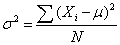Tags
Question

The formula for the variance in a population isTags
Question

The formula for the variance in a population is

?

Tags
Question

The formula for the variance in a population isIf you want to change selection, open document below and click on "Move attachment"

Subject 6. Measures of Dispersion
ons in the sample. However, the absolute value is difficult to work with mathematically. The variance is a measure of how spread out a distribution is. It is computed as the average squared deviation of each number from its mean. <span>The formula for the variance in a population is where: μ = the mean N = the number of scores When the variance is computed in a sample, the statistic(m = the mean of the sample) can be used

#### Summary

status measured difficulty not learned 37% [default] 0

No repetitions